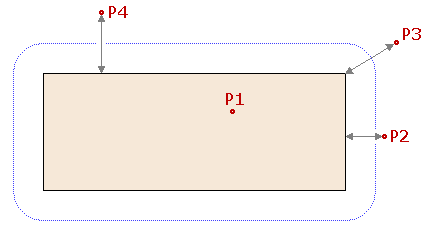The SDL Component Suite is an industry leading collection of components supporting scientific and engineering computing. Please visit the SDL Web site for more information....## DistFromRect

 Unit: SDL_math1 Class: None Declaration: function DistFromRect (RefPos: TPoint; Rectangle: TRect): integer;

The function DistFromRect calculates the distance of the reference point RefPos from a rectangular region given by the parameter Rectangle. The function returns a zero value if the reference point is within the rectangle, otherwise the distance from the nearest element of the rectangle (either a corner or an edge) is returned.

The following figure shows the distances of various reference points. The point P1 has zero distance (it is located within the rectangle), the distance of the point P2 is measured to the right edge of the rectangle, the distance of point P4 to the top edge, and the distance of P3 is measured to the upper right corner of the rectangle. The dotted blue line is a line of a constant distance from the rectangle.Last Update: 2017-Sep-11